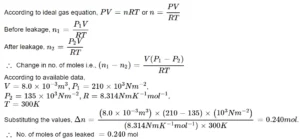# States of Matter Class 11 NumericalsStates of Matter Class 11 Numericals

States of Matter Class 11 Numericals

Que 1. Calculate the temperature of 4.0 moles of gas occupying 5 dm3 volume at 3.32 bar. (R= 0.083 bar dm3 k-1 mol-1).
Ans 1. PV = nRT
T = PV/nR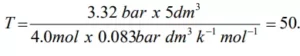Que 2. A sample of gas occupies 100 dm3 at 1 bar pressure and at 00C. If the volume of the gas is reduced to 5 dm3 at the same temperature, what additional pressure must be applied?
Ans 2.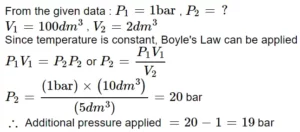Que 3. A mixture of dihydrogen and dinitrogen at 1 bar pressure contains 20% by weight of H2. Calculate the partial pressure of H2.
Ans 3. The mixture of H2 and O2 contains 20% H2 means 20 gm H2 & 80 gm O2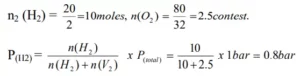Que 4. A certain amount of gas at 27 0C and bar pressure occupy a volume of 25 m3. If the pressure is kept constant and the temperature is raised to 77 0C, what will be the volume of the gas?
Ans 4. We know,
V1​T1 ​= V2​T2
V1 ​= 25 m3
T1​ = 27 0C = 300 K
T2 ​ = 77 0C = 350 K
Hence,
V2 = 25 × 350/300
V2= 5 × 35/6
V2 = 175/6
V2 = 29.1 dm3

Que 5. An O2 cylinder has 10 L O2 at 200 atm. It patient takes 0.50 ml of O2 at 1 atm in one breath at 37 0C. How many breaths are possible?
Ans 5. 10 L at 200 atm = ? L at 1 atm
P1V1 = P2V2
200 × 10 = 1 × V2
V2 = 2000 L,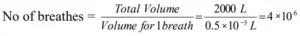Que 6. An open vessel at 27 0C is heated until three-fifth of the air has been expelled. Assuming that the volume of air is constant. Find the temperature to which vessel has been heated.
Ans 6. We know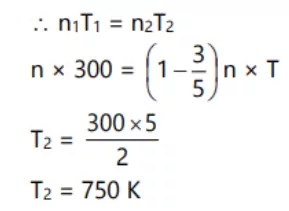Que 7. Calculate the pressure exerted by 110 g of carbon dioxide in a vessel of 2 I. capacity at 37 0C. Given that the van der Waals constants are a = 3.59 L2 atm mol-2 and b = 0.0427 L mol-1. Compare the value with the calculated value if the gas were considered ideal.
Ans 7. According to van der Waals equation,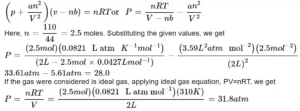Que 8. A vessel of volume 8 × 10-3 contains an ideal gas at 300 K and 210 kPa. The gas is allowed to leak till the pressure falls to 135 kPa. Calculate the amount of the gas leaked assuming the temperature to remain constant.
Ans 8. There is no change in the volume and temperature of the gas during leakage. However, the number of moles of the gas or its mass changes.